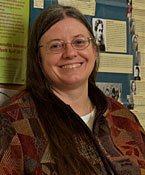# Dr. Fredricka Bennett

Professor, MathematicsEmail
Phone
(413) 662-5364
Office
Bowman Hall Rm 105B

## Education

Ph.D University of Massachusetts-
Amherst, MA

## Courses Taught

Mathematics for the Liberal Arts

Calculus I

Introduction to Statistics

Set Theory and Mathematical Logic

Linear Algebra

Linear Programming

How to Teach Mathematics

Calculus II, Calculus III

Probability Theory

Statistical Analysis

Graph Theory

Geometry

Game Theory

Number Theory

Algebraic Structures

Linear Algebra II

Computability and Complexity

History of Mathematics

Differential Equations

Calculus IV Topology

Complex Variables Operations Research Real Analysis

Cryptology

Mathematical Modeling

Issues and Trends in Mathematics Education

First Year Seminar - Science and Technology Problem Solving and Numeration

Data Analysis.

### Research/ Creative Interests

Graph Theory, History of Mathematics, Mathematical Statistics, Mathematics Education, Mathematical Modeling of the Environment, and Financial Mathematics